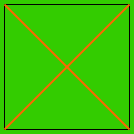Name: Michelle Who is asking: Teacher Level: Middle Question: Hey. I want to have my students create nets for pyramids and I need to know how to find the correct range of degrees for the interior congruent angles of the isosceles triangular faces. For example, I know for a square-based pyramid that 77 degrees will work; however, I know other angle measures will also work. I'm just not sure how to find the minimum degree measure to have the net actually "work". I'm assuming the maximum would be 89 degrees, although that would make for a very tall pyramid. Is there a formula that allows me to calculate the range of degrees that would work given the base of the pyramid (e.g. square, pentagonal, hexagonal, etc.)? Thanks for your help. Michelle Hi Michelle, Imagine that you draw a square on the ground and drive a peg at each of the four corners. Tie an elastic band across each of the two diagonals to form the diagram below.This forms a "pyramid" of zero height and each of the four triangles has interior congruent angles of 45o. Now grab the two elastic bands where they cross an lift them vertically off the ground to form a pyramid. As the height of the pyramid increases, the interior congruent angles on each side also increase. The taller the pyramid, the closer these angles are to 90o. Hence the interior congruent angles can have any value greater than 45o and less than 90o. The same idea will work for any regular polygon as the base. Draw a line from each vertex to the center of the polygon to form triangles with the interior congruent angles having minimum possible angle measurement. Cheers, Penny Go to Math Central